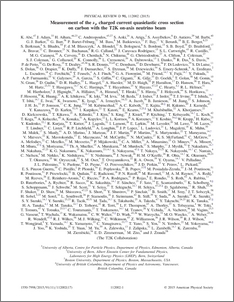Measurement of the $ν_μ$ charged current quasi-elastic cross-section on carbon with the T2K on-axis neutrino beam

, T2K Collaboration (2015) Measurement of the $ν_μ$ charged current quasi-elastic cross-section on carbon with the T2K on-axis neutrino beam. Physical Review D – Particles and Fields, 91 (11). ISSN 1550-7998

Other (pdf)
1503.07452v1 - Accepted VersionPreview
PDF (PhysRevD.91.112002)
PhysRevD.91.112002.pdf - Published Version

Abstract

We report a measurement of the $\nu_\mu$ charged current quasi-elastic cross-sections on carbon in the T2K on-axis neutrino beam. The measured charged current quasi-elastic cross-sections on carbon at mean neutrino energies of 1.94 GeV and 0.93 GeV are $(11.95\pm 0.19(stat.)_{-1.47}^{+1.82} (syst.))\times 10^{-39}\mathrm{cm}^2/\mathrm{neutron}$ and $(10.64\pm 0.37(stat.)_{-1.65}^{+2.03} (syst.))\times 10^{-39}\mathrm{cm}^2/\mathrm{neutron}$, respectively. These results agree well with the predictions of neutrino interaction models. In addition, we investigated the effects of the nuclear model and the multi-nucleon interaction.

Item Type:
Journal Article
Journal or Publication Title:
Physical Review D – Particles and Fields
17 pages, 21 figures
Subjects:
Departments:
ID Code:
73884
Deposited By:
Deposited On:
18 Jun 2015 05:55
Refereed?:
Yes
Published?:
Published